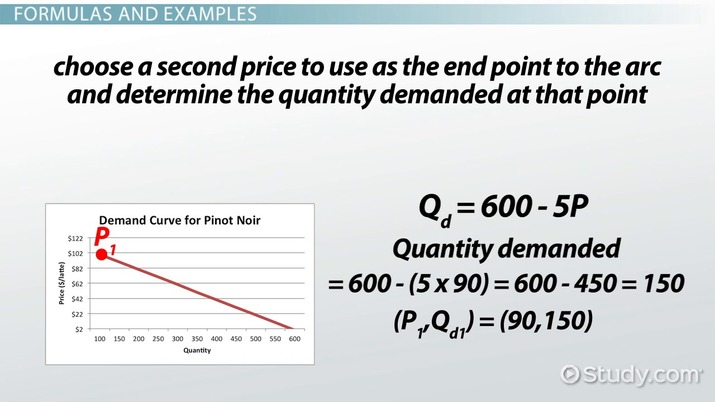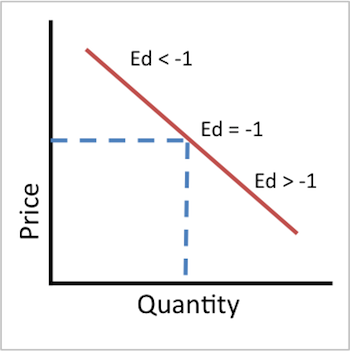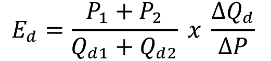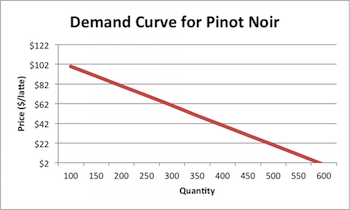# Elastic Demand: Definition, Formula & Examples

Lesson Transcript
Instructor: Kallie Wells

Kallie has a B.S. in Agribusiness and minor in Statistics from California Polytechnic State University, San Luis Obispo and M.S. in Agricultural and Resource Economics from University of California, Davis. She has extensive experience designing and performing economic analysis of wholesale energy markets and investigations of market participant behavior within these markets.

In this lesson, you will be introduced to the concept of an elastic demand and how to determine if the demand is elastic. Two methods will be presented along with examples.

## What Is Elastic Demand?

An elastic demand curve is one where the quantity demanded of a given good is sensitive to changes in price. For example, if airline tickets to Maui increased by 10% and the amount of people buying tickets dropped by more than 10%, then airline tickets to Maui would be considered to have an elastic demand. Compare this to if milk increased by 10%, but there was only a 2% drop in the amount of gallons sold; the change in price didn't significantly impact the amount of milk sold. More specifically, a demand curve is considered elastic if a 1% change in price results in more than a 1% change in quantity demanded.

The following image illustrates an important concept with elasticity. Elasticity is not constant along a linear line like the slope of the line. Elasticity changes as you move along the line because it all is based on percent changes. A 10% change at \$1 is significantly less than a 10% change at \$5. To accurately determine if a demand curve is elastic, you have to calculate the elasticity at a given price. The only elastic demand curve that has a constant elasticity is a horizontal line.An error occurred trying to load this video.

Try refreshing the page, or contact customer support.

Coming up next: Inelastic Demand: Definition & Examples

### You're on a roll. Keep up the good work!

Replay
Your next lesson will play in 10 seconds
• 0:00 What Is Elastic Demand?
• 1:15 Formulas and Examples
• 6:15 Lesson Summary
Save Save

Want to watch this again later?

Timeline
Autoplay
Autoplay
Speed Speed## Formulas And Examples

Price elasticity of demand can be calculated using the arc or price point method. We will walk through an example using both methods. Essentially, both methods are trying to determine what the change in quantity demanded will be if there is a change in price. The more sensitive, i.e. more change, in quantity demanded, the more elastic the demand curve. Think of something that you treat yourself to. If that price increases, you would probably treat yourself less often. If the price goes up 1% and you decrease the amount you buy by more than 1%, it is considered to have an elastic demand.

Arc Price Elasticity Formula• Where P1 and P2 are two price points on the demand curve.
• Qd1 and Qd2 are the quantity demanded given P1 and P2.
• Delta Qd = Qd2 - Qd1
• Delta P = P1 - P2

This method is used primarily when you don't have a formula for the demand curve, or you aren't familiar with taking derivatives of equations. The arc method essentially takes the average elasticity between the two points chosen. The more curved the demand is between the two points, the more inaccurate the estimate.

Arc Price Elasticity Example:

The following graph shows the demand curve for Pinot Noir after the movie Sideways. The demand curve is Qd = 600 - 5P.Using the arc method, determine the price elasticity at \$90/bottle.

Given the demand equation above, first determine the quantity demanded at \$90/bottle.

Quantity demanded = 600 - (5 x 90) = 600 - 450 = 150
(P1,Qd1) = (90,150)

Now choose a second price to use as the end point to the arc and determine the quantity demanded at that point. The closer the second point is to the original price point, the less inaccurate the estimate will be. Let's pick \$31/bottle as P2.

Quantity demanded = 600 - (5 x 91) = 600 - 455 = 145
(P1,Qd1) = (91,145)

Using the formula from earlier:

To unlock this lesson you must be a Study.com Member.

### Register to view this lesson

Are you a student or a teacher?